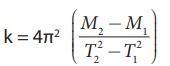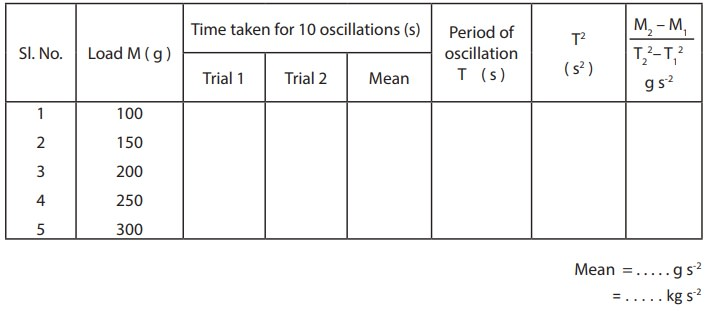Home | | Physics 11th std | Spring Constant of a Spring

# Spring Constant of a Spring

To determine the spring constant of a spring by using the method of vertical oscillations

SPRING CONSTANT OF A SPRING

## AIM

To determine the spring constant of a spring by using the method of vertical oscillations

## APPARATUS REQUIRED

Spring, rigid support, hook, 50 g mass hanger, 50 g slotted masses, stop clock, metre scale, pointer

## FORMULA

Spring constant of the spring kwhere

M1, M2 â†’ selected loads in kg

T1, T2 â†’ time period corresponding to masses M1 and M2 respectively in second

## DIAGRAM## PROCEDURE

Â·           A spring is firmly suspended vertically from a rigid clamp of a wooden stand at its upper end with a mass hanger attached to its lower end. A pointer fixed at the lower end of the spring moves over a vertical scale fixed.

Â·         A suitable load M (eg; 100 g ) is added to the mass hanger and the reading on the scale at which the pointer comes to rest is noted. This is the equilibrium position.

Â·         The mass in the hanger is pulled downward and released so that the spring oscillates vertically on either side of the equilibrium position.

Â·         When the pointer crosses the equilibrium position a stop clock is started and the time taken for 10 vertical oscillations is noted. Then the period of oscillation T is calculated.

Â·         The experiment is repeated by adding masses in steps of 50 g to the mass hanger and period of oscillation at each time is calculated.

Â·         For the masses M1 and M2 ( with a difference of 50 g ), if T1 and T2 are the corresponding periods, then the value M2 â€“ M1 / T22 â€“ T12 is calculated and its average is found.

Â·         Using the given formula the spring constant of the given spring is calculated.

## OBSERVATIONS## CALCULATION

Spring constant of the spring k## RESULT

The spring constant of the given spring k = . . . . . . . . . . . . kg s-2

Tags : Physics Laboratory Practical Experiment , 11th Physics : Practical Experiment
Study Material, Lecturing Notes, Assignment, Reference, Wiki description explanation, brief detail
11th Physics : Practical Experiment : Spring Constant of a Spring | Physics Laboratory Practical Experiment